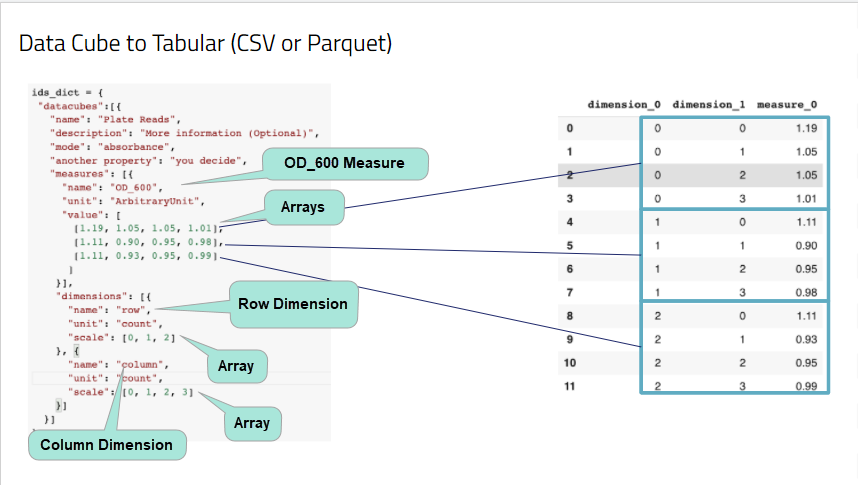Once data has been processed, the data is stored in a data cube, which is a multi-dimensional array of complex values. Having a cursory understanding of data cubes is important as you review the data because it will help you better understand SQL query results.

### Cube Concepts: Measures and Dimensions

Cube data is grouped into different dimensions, indexed, and have precomputed queries frequently performed. Because of this, data cubes speed query processing.

There are two dimensions groupings:

• Measure - Provides information that you can perform mathematical operations on. For example, temperature or humidity. Measure dimensions (often referred to as simply "measures") Measures are dependent variables.
• Dimensions - Typically qualitative, such as a row or column number. Often referred to as simply "dimensions", these are independent variables. Both measures and dimensions are arrays. An array is an ordered collection of elements.

Let's take a look at an example to better understand these concepts.

``````{
"datacubes": [
{
"mode": "absorbance",
"another_property": "you decide",
"measures": [
{
"name": "OD_600",
"unit": "ArbitraryUnit",
"value": [
[1.19, 1.05, 1.05, 1.01],
[1.11, 0.90, 0.95, 0.98],
[1.11, 0.93, 0.95, 0.99]
]
}
],
"dimensions": [
{
"name": "row",
"unit": "count",
"scale": [0, 1, 2]
},
{
"name": "column",
"unit": "count",
"scale": [0, 1, 2, 3]
}
]
}
]
}
``````

RAW data from a spectrophotometer that contains the following variables. Which are measures and dimensions?

• Optical density at 600 nm wavelength
• Row of the plate well
• Column of the plate well

In this example, the row and columns for the well are descriptive; they simply indicate where the samples were taken from. These are independent variables. Row of the plate well and column of the plate well are dimensions.

The optical density at 600 nm wavelength is a dependent variable (the data depends on the location of the well). The optical density is a measure.

A few other examples appear in the table below.

Example

Measure(s)

Dimension(s)

Chromatogram

1. Detector Intensity
1. Wavelength
2. Retention Time

Weather

1. Temperature
2. Humidity
1. Longitude
2. Latitude

1. Absorbance
2. Concentration
1. Row Position
2. Column Position

### Mapping the Cube to Table Structure

Note that data cubes are often visualized as a "rubik's cube-like structure" but are typically stored in SQL tables. Measures and dimensions are mapped to table rows, columns, and values. To better understand this, let’s take a look at the following figure.In the figure, there are two dimensions and one measure. Their names are “row”, “column” and “OD_600”.

Table column headers are the measures and dimensions with a number appended to them. The number simply indicates the numerical order, starting with the number 0, of the dimension or measure in the JSON file. Since there are two dimensions and one measure, the column headers are dimension_0 (row dimension), dimension_1 (column dimension), and measure_0 (OD_600 measure).

Each dimension has an array. An array is simply an ordered list. In this example, the measure has three arrays. Each array contains elements (values).

Values in the dimension and measure arrays are collated based on the order in which the element is listed in the array. For example in the second measure array [1.11, 0.90, 0.95, and 0.98] the elements are in these positions:

Array Element Position

Measure Value

0 (first)

1.11

1 (second)

.90

2 (third)

.95

3 (fourth)

.98

The position of elements in an array are important because values in each row of the table collated based on the position of the elements across different arrays.